# Maharashtra Board 10th Class Maths Part 1 Practice Set 4.1 Solutions Chapter 4 Financial Planning

Balbharti Maharashtra State Board Class 10 Maths Solutions covers the Practice Set 4.1 Algebra 10th Class Maths Part 1 Answers Solutions Chapter 4 Financial Planning.

## Practice Set 4.1 Algebra 10th Std Maths Part 1 Answers Chapter 4 Financial Planning

Financial Planning Class 10 Practice Set 4.1 Question 1.
‘Pawan Medical’ supplies medicines. On some medicines the rate of GST is 12%, then what is the rate of CGST and SGST?
Solution: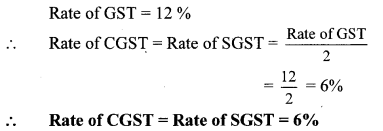Question 2.
On certain article if rate of CGST is 9% then what is the rate of SGST? and what is the rate of GST?
Solution:
Rate of CGST = 9%
But, rate of SGST = rate of CGST
∴ Rate of SGST = 9%
Rate of GST = Rate of SGST + Rate of CGST = 9% + 9%
∴ Rate of GST = 18%

Financial Planning Class 10 Question 3.
‘M/s. Real Paint’ sold 2 tins of lustre paint and taxable value of each tin is ₹ 2800. If the rate of GST is 28%, then find the amount of CGST and SGST charged in the tax invoice.
Solution:
Taxable value of 1 tin = ₹ 2800
∴ Taxable value of 2 tins = 2 × 2800
= ₹ 5600
Rate of GST = 28 %
∴ Rate of CGST = Rate of SGST = 14 %
CGST = 14% of taxable value 14
= $$\frac { 14 }{ 100 }$$ × 5600
∴ CGST = ₹ 784
∴ SGST = CGST = ₹ 784
∴ The amount of CGST and SGST charged in the tax invoice is ₹ 784 each.

Question 4.
The taxable value of a wrist watch belt is 7 586. Rate of GST is 18%. Then what is price of the belt for the customer?
Solution:
Taxable value of wrist watch belt = ₹ 586
Rate of GST = 18%
∴ GST = 18% of taxable value
= $$\frac { 18 }{ 100 }$$ × 586
∴ GST = ₹ 105.48
∴ Amount paid by customer = Taxable value of wrist watch belt + GST
= 586+ 105.48
= ₹ 691.48
∴ The price of the belt for the customer is ₹ 691.48.

Question 5.
The total value (with GST) of a remote-controlled toy car is ₹ 1770. Rate of GST is 18% on toys. Find the taxable value, CGST and SGST for this toy-car.
Solution:
Let the amount of GST be ₹ x.
Total value of remote controlled toy car = ₹ 1770
∴ Taxable value of remote controlled toy car = ₹ (1770 – x)
Now, GST = 18% of taxable value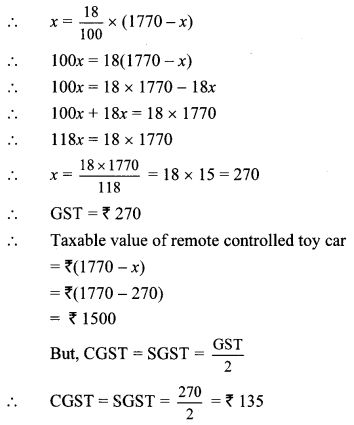∴ Taxable value of toy car is ₹ 1500 and CGST and SGST for it is ₹ 135 each.

Question 6.
‘Tiptop Electronics’ supplied an AC of 1.5 ton to a company. Cost of the AC supplied is ₹ 51,200 (with GST). Rate of CGST on AC is 14%. Then find the following amounts as shown in the tax invoice of Tiptop Electronics.
i. Rate of SGST
ii. Rate of GST on AC
iii. Taxable value of AC
iv. Total amount of GST
v. Amount of CGST
vi. Amount of SGST
Solution:
i. Rate of CGST = 14%
But, Rate of SGST = Rate of CGST
∴ Rate of SGST = 14%

ii. Rate of GST on AC
= Rate of SGST + Rate of CGST
= 14% + 14% = 28%
∴ Rate of GST on AC is 28%.

iii. Let the cost (Taxable value) of AC be ₹ 100.
Given, GST = 28%
∴ The cost of AC with GST is ₹ 128.
For the total value of ₹ 128, the taxable value is ₹ 100.
For the total value of ₹ 51200, let the taxable value be ₹ x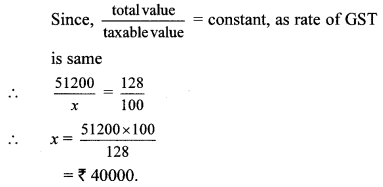∴ Taxable value of AC is ₹ 40,000.

iv. Total amount of GST = 28% of taxable value
= $$\frac { 28 }{ 100 }$$ × 40000
= ₹ 11,200
∴ Total amount of GST is ₹ 11,200.

∴ Amount of CGST is ₹ 5600.vi. Amount of SGST = Amount of CGST
= ₹ 5600
Amount of SGST is ₹ 5600.

Question 7.
Prasad purchased a washing-machine from ‘Maharashtra Electronic Goods’. The discount of 5% was given on the printed price of ₹ 40,000. Rate of GST charged was 28%. Find the purchase price of washing machine. Also find the amount of CGST and SGST shown in the tax invoice.
Solution:
Printed price of washing machine = ₹ 40,000
Rate of discount = 5%
Amount of discount = 5% of printed price
= $$\frac { 5 }{ 100 }$$ × 40000 = ₹ 2000
∴ Taxable value = Printed price – Discount
= 40000 – 2000 = ₹ 38000
Rate of GST = 28%
∴ Rate of CGST = 14% and
Rate of SGST = 14%
CGST = 14% of taxable value
= $$\frac { 14 }{ 100 }$$ × 38000
∴ CGST = ₹ 5320
∴ CGST = SGST = ₹ 5320
Purchase price of washing machine
= Taxable value + CGST + SGST
= 38000 + 5320 + 5320
= ₹ 48,640
∴ Purchase price of washing machine is ₹ 48640. Amount of CGST and SGST in tax invoice is ₹ 5320 each.

Question 1.
Observe the given bill and fill in the boxes with the appropriate number. (Textbook pg. no. 82 and 83)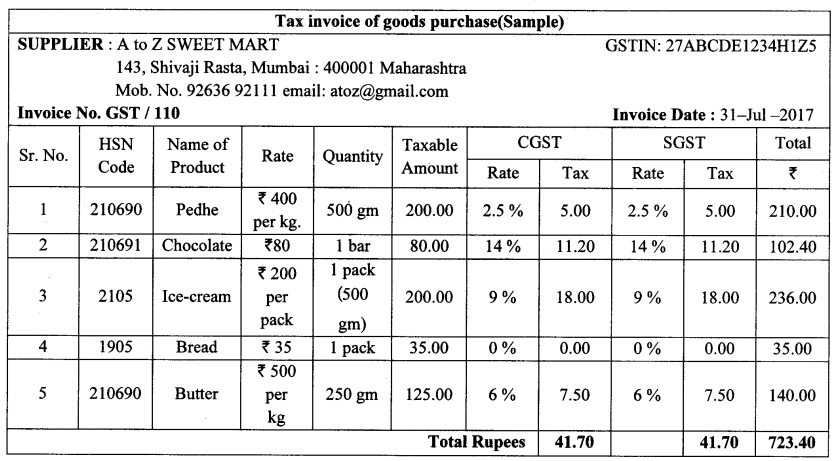Solution:
i. Price of 1 kg of Pedhe is ₹ 400, therefore cost of 500 gm. of Pedhe is ₹ 200.
CGST for pedhe at the rate of 2.5% is ₹  and SGST at the rate of [2.5| % is ₹ 5.00. It means that the rate of GST on Pedhe is 2.5% + 2.5% = 5% and hence the total GST is ₹ 10.
ii. The rate of GST on chocolate is  % and hence the total GST is ₹ [22.40]
iii. Rate of GST on Ice-cream is  %, hence the total cost of ice-cream is ₹ 236
iv. On butter CGST rate is  % and SGST rate is also  %. So GST rate on butter is %.

Question 2.
Fill in the blanks with the help of given information for the table given below. (Textbook pg. no. 83)
Solution:Question 3.
Make a list of ten things you need in your daily life. Find the GST rates with the help of GST rate chart given in the textbook, news papers or books, internet, or the bills of purchases. Verify these rates with the list prepared by your friends. (Textbook pg. no. 85)
Solution: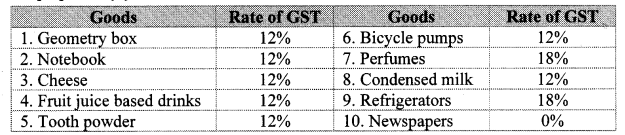Question 4.
Make a list of ten services and their GST rates as per activity 1. (e.g. Railway and ST bus booking services etc.) You can also collect service bills and complete the given information (Textbook pg. no. 85)
Solution: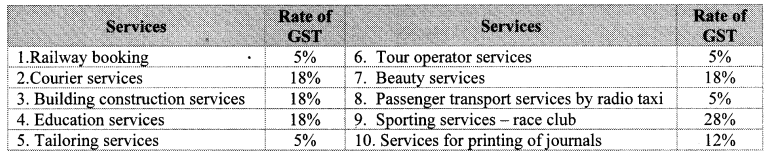Question 5.
Complete the given table by writing remaining SAC and HSN codes with rates and add some more items in the list. (Textbook pg, no. 85)
Solution: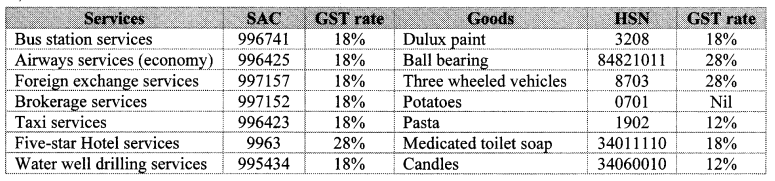[Note : The above Activities has many answers students may write answers other than the ones given]

Scroll to Top